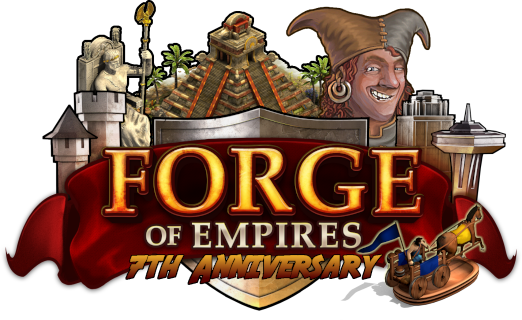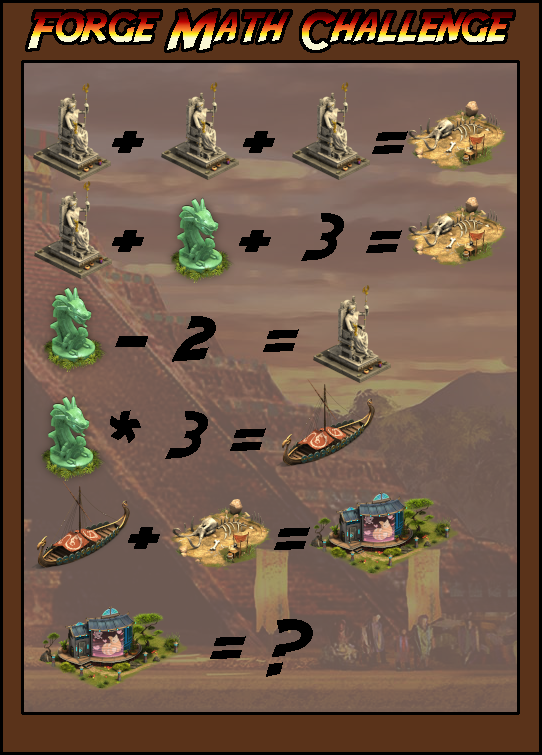# Contest day 6

Status
Not open for further replies.

Zeus = 5

5+5+5=15
5+7+3=15
7-2=5
7*3=21
15+21=36

36

Result = 36

zeus=5
relic=7
viking boat=21
so ansver is 36

36

36

#### Blue Glass

##### Private
5 + 5 + 5 = 15 Zeus: 5 Jade relic: 7 Skeleton incident: 15 Boat incident : 21 VR House: 36
5 + 7 + 3 = 15
7 - 2 = 5
7 x 3 = 21
21 + 15 = 36
36

36

36

5 + 5 + 5 = 15
5 + 7 + 3 = 15
7 - 2 = 5
7 * 3 = 21
21 + 15 = 36

#### GoldRain69

##### Private#### Necro

##### Private
the blue building (whatever it is as never seen one) = 36 (that is bearing in mind that the strange/unusual symbol between the jade lion statue and the number 3 on the 4th line is suppose to be a multiplication indictaor)……. so I re-iterate that the answer is 36....

• 5+5+5=15
• 5+7+3=15
• 7-2=5
• 7x3=21
• 21+15=36

#### Old White Rabbit

##### PrivateGreetings, Queens and Kings!

2018 brought the Reconstruction Mode to the game, which was one of the most requested features of the game. The research "Mathematics" was needed to unlock it, but now it is on you to use some mathematics to solve the following puzzle!For this contest, 7 players can win 250 goods of your choice! Have fun calculating!

Please submit the correct answer until April 28, 2019. We will pick the 7 lucky winners from all correct answers!Sincerely,

18

45

36

36

#### zaini3

##### Private
Ans = 36

Status
Not open for further replies.